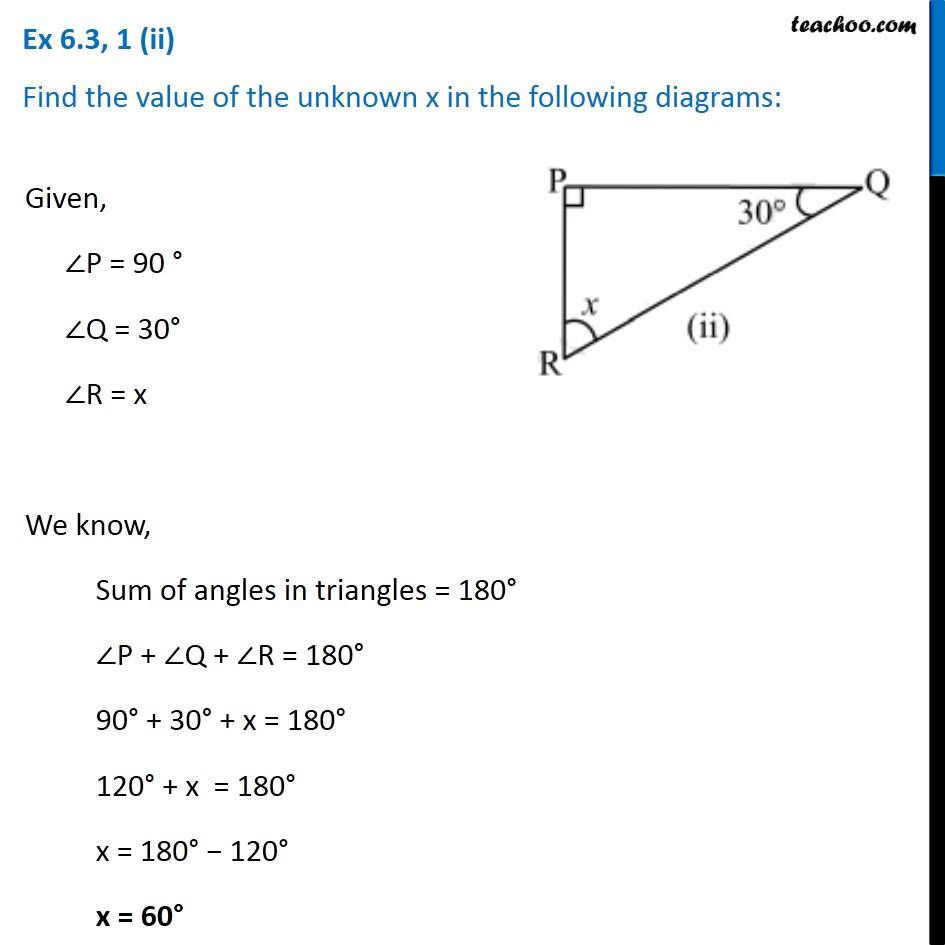Ex 6.3

Chapter 6 Class 7 Triangle and its Properties
Serial order wiseLearn in your speed, with individual attention - Teachoo Maths 1-on-1 Class

### Transcript

Ex 6.3, 1 (ii) Find the value of the unknown x in the following diagrams: Given, ∠P = 90 ° ∠Q = 30° ∠R = x We know, Sum of angles in triangles = 180° ∠P + ∠Q + ∠R = 180° 90° + 30° + x = 180° 120° + x = 180° x = 180° − 120° x = 60°